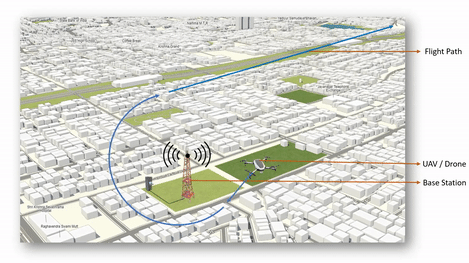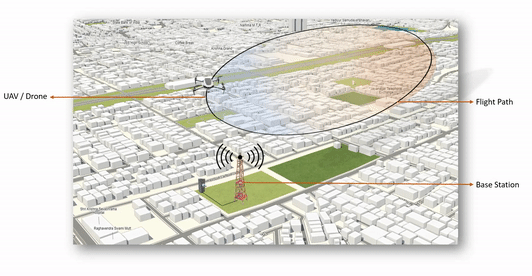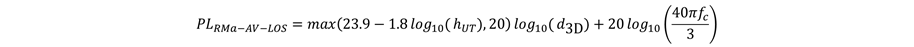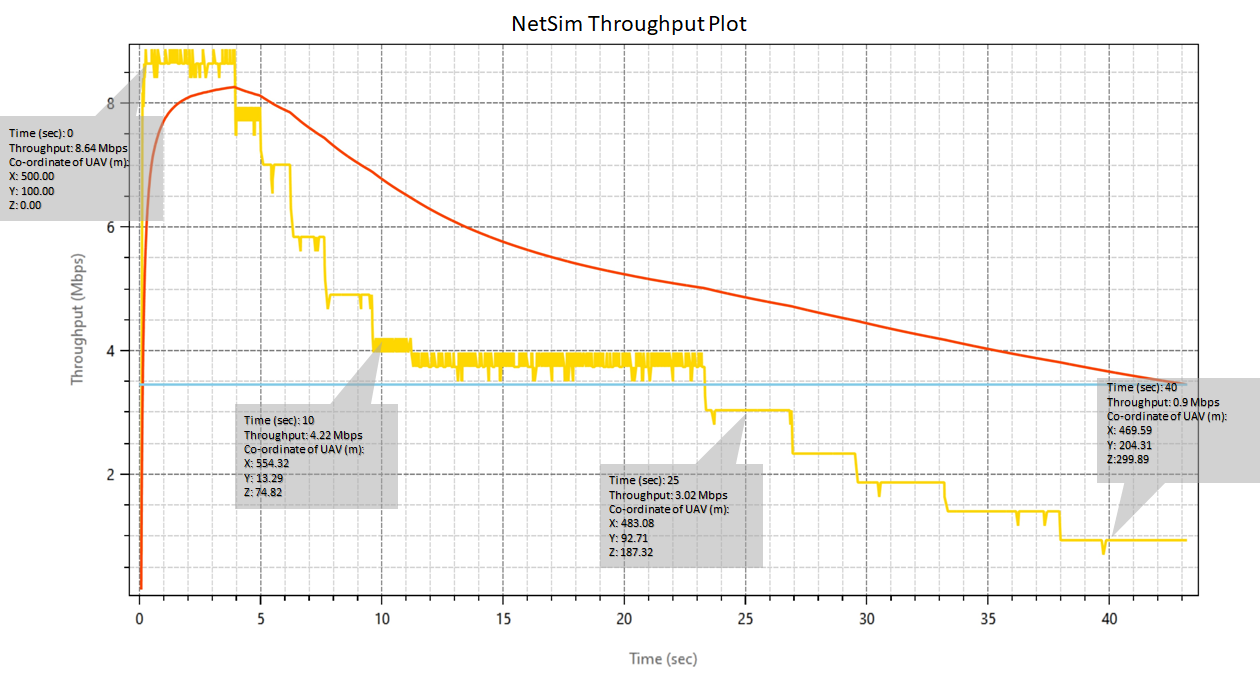Applicable Versions NetSim Standard NetSim Pro

 Applicable Releases v12.2 v13

 MATLAB Version R2019b or above MATLAB Toolbox MATLAB, Simulink, Robotics and System Toolbox, UAV Toolbox File Exchange Project of MATLAB (Only for < R2020a) Robotics System Toolbox UAV Library Visual Studio Community Edition

### Objective

Performance analysis of UAV - Base station network communications. This involves interfacing NetSim and UAV toolbox of MATLAB

• For Each UE in NetSim, a UAV is instantiated in MATLAB as per the UE (UAV) ID.
• MATLAB calculates the flight path and passes the Mobility information to NetSim.
• In NetSim UE movement is modelled as per MATLAB UAV co-ordinates
• Pathloss calculations done in NetSim per 36.777

### NetSim UAV Simulation modelUAV Takes and moves away from gNB UAV flies in a circular pattern over the gNB Pathloss calculations done in NetSim per 36.777  for Rural Macrohttps://tetcos.freshdesk.com/support/solutions/articles/14000099351-how-to-clone-netsim-fileexchange-project-repositories-from-github

Other tools such as GitHub Desktop, SVN Client, Sourcetree, Git from the command line, or any client you like to clone the Git repository.

v12: https://github.com/NetSim-TETCOS/LTE-UAV-Simulation_v12.2.git

`Note: It is recommended not to download the project as an archive (compressed zip) to avoid incompatibility while importing workspaces into NetSim.`

### Running the NetSim scenario with UAV Based Mobility

• Before opening, make sure that you have replaced the XML file in the NetSim Installation directory mentioned above and then Open Example saved in the workspace.
• Make sure that all UAV/Drone/UE's are UAV Mobility enabled and the path to MATLAB files that you downloaded with the attachment
• Also, make sure that you provide the path using single inverted commas. ('<Matlab Files Path>')
• Run the simulation with Play and record animation in order to have both Animations simultaneously

#### Case 1: UAV Drone Takeoff - Application throughput variation with Drone mobility

• NetSim Plot: Application Throughput vs. Time• MATLAB Flight Path#### Case 2: UAV Drone rotation around LTE-eNB

• NetSim Plot: Application Throughput Vs. Time• MATLAB Flight Path### Code modifications

Files attached and their modifications are explained below

1. LTENR_PropagationModel.c - Added LTE-UAV propagation model for Rural macro as per 3GPP 36.777 Standard

2. UAVBasedMobility.c - A new created added to the Mobility Project. The interface with MATLAB happens here to get co-ordinates from MATLAB and to pass it to NetSim

Upon interfacing NetSim with MATLAB the following tasks are performed during simulation start:

• MATLAB Engine process is initialized
• MATLAB Desktop window is loaded

Upon simulating a network created in NetSim the following tasks are performed periodically:

• Appends the readings to the packet payload as packets are formed

During the Simulation, the SIMULINK Model is started and paused several times for NetSim and SIMULINK simulations to run synchronously. The X, Y and Z coordinates obtained from SIMULINK are read from MATLAB workspace and given as input to NetSim's Mobility model. In this example, coordinates are taken every one second and updated to the device mobility.

### Output/Metrics specific to this example

• NetSim Animation: Mobility of the devices configured in NetSim is given as input from MATLAB
• Pathloss is implemented as per 36.777 Standard
• NetSim Plot Results: Throughput analysis as the UAV moves away from the gNB

### Modifications done to NetSim Source codes:

#### Files:

• Mobility.c,
• Mobility.h,
• Mobility.vcxproj (Project file)

#### Sections of source code modified

• Mobility.c
• fn_NetSim_Mobility_Init(): call to init_uav() function
• fn_NetSim_Mobility_Run(): Call to uav_run() function
• fn_NetSim_Mobility_Finish()
• Mobility.h
• MATLAB Engine variable - Used to initiate and interact with MATLAB Engine process
• Mobility.vcxproj - This is a Visual Studio project file used to load and manage the source codes related to the Mobility in NetSim
• path to MATLAB application
• path to MATLAB include directory
• path to MATLAB lib directory
• information related to dependent MATLAB library files
• UAVBasedMobility.c
• init_uav():  Initializes MATLAB, Loads SIMULINK Model, starts and pauses SIMULINK simulation, and initializes the UAV devices in MATLAB to start simulation along with NetSim's simulation.
• uav_run(): Starts NetSim and MATLAB simulation simultaneously and gets the co-ordinates from MATLAB workspace for every step size set in NetSim.
• uavcorr():  Function to get co-ordinates from MATLAB.

### Procedure to setup MATLAB for this example

• Create a system environment variable with name as "MATLAB_PATH" and value as "<path of MATLAB Root directory>"Note: The procedure to create an environment variable and the path of the MATLAB root directory will vary based on the Windows OS version and the MATLAB software version respectively.

### Procedure to setup NetSim for this example

• Clone the project using the Git-Hub link provided with the beginning of this article. And here is the procedure on How to clone NetSim File Exchange project repositories from GitHub.
• Import the workspace to NetSim. Refer Chapter 4, Section 4.9 of User Manual.
• Place MATLAB files in a system location with both read and write permissions.
• Backup and Replace GenerlProperty.xml file provided in NetSim-Files folder in the cloned project to <NetSim-Installation-Directory>/Docs/XML/Properties folder.
• Backup and Replace Configuration.xsd file provided in the NetSim-Files folder in the cloned project to <NetSim-Installation-Directory>/bin/bin_x64 folder.
• Open code from NetSim Home Screen. (NetSim Home Screen > Open Simulation > Open code)
• Rebuild the code